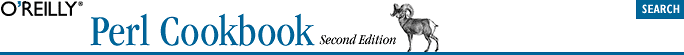home | O'Reilly's CD bookshelfs | FreeBSD | Linux | Cisco | Cisco Exam## 4.18. Randomizing an Array

### 4.18.3. Discussion

Shuffling is a surprisingly tricky process. It's easy to write a bad shuffle:

```sub naive_shuffle {                             # DON'T DO THIS
for (my \$i = 0; \$i < @_; \$i++) {
my \$j = int rand @_;                    # pick random element
(\$_[\$i], \$_[\$j]) = (\$_[\$j], \$_[\$i]);    # swap 'em
}
}```

This algorithm is biased; the list's possible permutations don't all have the same probability of being generated. The proof of this is simple: take the case where we're passed a three-element list. We generate three random numbers, each of which can have three possible values, yielding 27 possible outcomes. There are only six permutations of the three-element list, though. Because 27 isn't evenly divisible by 6, some outcomes are more likely than others.

The List::Util module's shuffle function avoids this bias to produce a more randomly shuffled result.

If all you want to do is pick one random element from the array, use:

`\$value = \$array[ int(rand(@array)) ];`